# Converting Units Of Measure Worksheet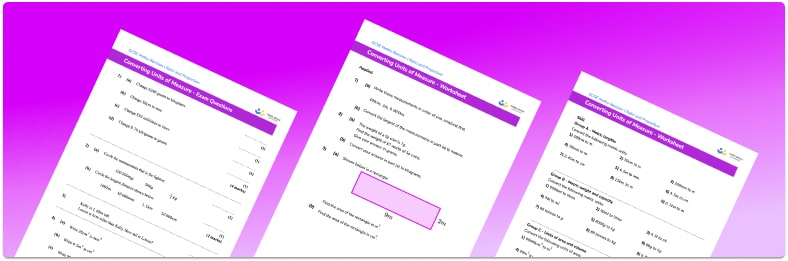• Section 1 of the converting units of measure worksheet  contains 27 skills-based time unit conversion questions, in 3 groups to support differentiation
• Section 2 contains 3 applied converting units of measure questions with a mix of word problems and deeper problem solving questions
• Section 3 contains 4 foundation and higher level GCSE exam style converting units of measure questions
• Answer sheets and a mark scheme for all converting units of measure questions are provided
• Questions follow variation theory with plenty of opportunities for students to work independently at their own level
• All teaching resources created by fully qualified expert secondary maths teachers
• Suitable for GCSE maths revision for AQA, OCR and Edexcel exam boards

• This field is for validation purposes and should be left unchanged.

You can unsubscribe at any time (each email we send will contain an easy way to unsubscribe). To find out more about how we use your data, see our privacy policy.

### Converting units of measure at a glance

Metric unit conversion involves converting between metric units of measurement, for example between centimetres and millimetres, which are metric units of length. Measurement conversion might be done to make the measurement easier to understand or in order to perform calculations.

We use metric measurement units for length, mass and capacity. To convert metric measurements, we need to know the conversion rates for different units . For example, the metric conversions for converting units of length are 1km=1000m, 1m=100cm and 1cm=10mm . It can be helpful to look at a conversion chart which shows these values.

The metric system uses different prefixes to mean different things. Examples of these prefixes are milli, centi and kilo. Wherever milli is used, for example, it means 1000th of the unit, so 1 millilitres =0.001 litre and 1 millimetres =0.001 metre.

Converting metric units of area and volume is an extension of converting metric units of length. When converting between metric units of area, we need to square the conversion rate for the units of length.

For example, there are 10 millimetres in a centimetre so to convert from centimetres to millimetres we multiply by 10 . To convert from centimetres squared to millimetres squared we multiply by 10^2. Similarly, when converting between metric units of volume, we need to cube the conversion rate for the units of length.

Looking forward, students can then progress to additional conversion worksheets and other ratio and proportion worksheets, for example a ratio worksheet or a simplifying and equivalent ratios worksheet.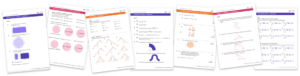For more teaching and learning support on Ratio and Proportion our GCSE maths lessons provide step by step support for all GCSE maths concepts.

## Related worksheets

Unit Conversion Worksheet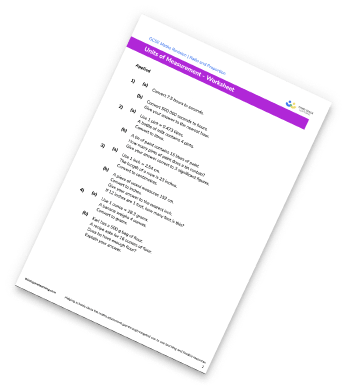Converting Units Of Area And Volume Worksheet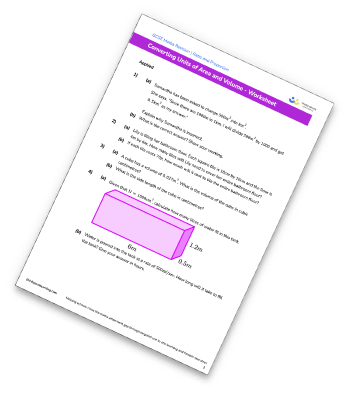Metric Conversion Worksheet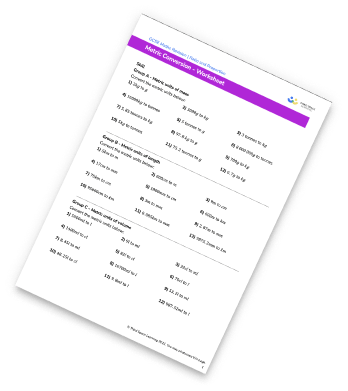Time Unit Conversion Worksheet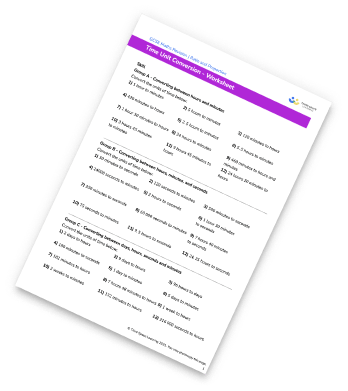## Do you have KS4 students who need more focused attention to succeed at GCSE?There will be students in your class who require individual attention to help them succeed in their maths GCSEs. In a class of 30, it’s not always easy to provide.

Help your students feel confident with exam-style questions and the strategies they’ll need to answer them correctly with our dedicated GCSE maths revision programme.

Lessons are selected to provide support where each student needs it most, and specially-trained GCSE maths tutors adapt the pitch and pace of each lesson. This ensures a personalised revision programme that raises grades and boosts confidence.

Find out more